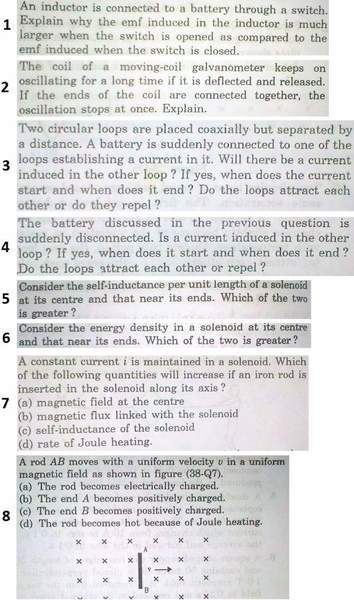# EMI quickies#1
No idea.

#2
Please someone explain me the question. From what I have understood, I think it’s because on connecting wires, it will form a closed loop and will induce an opposing EMF which will induce current in circuit and which will provide an opposing torque to the motion.

#3
Yes, start at instance battery is connected and continue to rise till infinity as current in first will also me maximum at infinity and will keep on increasing till then

#4
Same reason as third, current will be 0 at infinity

#5
Doesn’t inductance only depend on just geometry of solenoid? Then why will it change for ends?

#6
I guess at center as magnetic field lines are denser at center.

#7
The rod will magnetize so field and flux will increase, but answer is (a), (b), (c)

#8
Answer says that (d) is wrong, why? While electron will shift at B, they will collide and produce heat, right?

#### Attachments

ehild
Homework Helper
1. How does the current change with time after the switch is closed? What is the time derivative of the current at t=0? And how much is the change of the current when the switch is opened? How long can it last to open a switch?

ehild

For closing: i = io (1 - e-t/τ)
so di/dt = ioe-t/τ / τ
at t=0, i = io

For opening, i = io e-t/τ
di/dt = -io e-t/τ / τ
di/dt = -io / et/τ τ

As 1 > e-x > 0 for x>0

di/dt in opening is greater than one ... right?

ehild
Homework Helper
What is Io when the switch is closed? How is it related to the battery and the resistance in the circuit? There is always some resistance: the inner resistance of the battery, the resistance of the wires, the resistance of the coil (it is made of wire which has some resistance)

Can current flow at all when the switch is open? The equation you wrote refers to the case when the battery is short-circuited at t=0, at the current is io at that instant. It is not breaking the circuit.

ehild

8. no reason it will not heat up, unless it has no resistance or there is only 1 correct answer, in which case end A will become positively charged answer (C) (because of Lorenz force).

SammyS
Staff Emeritus